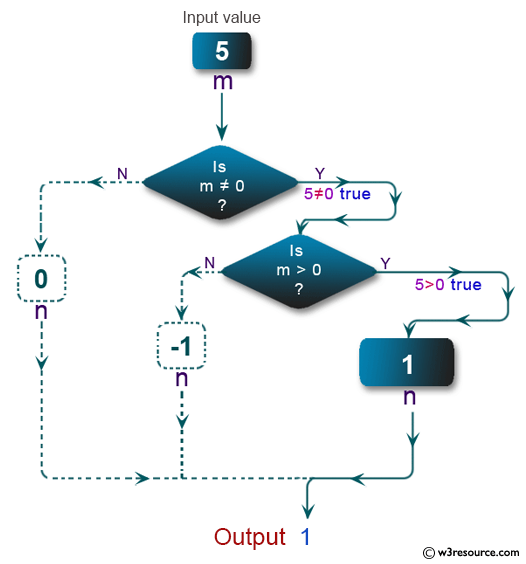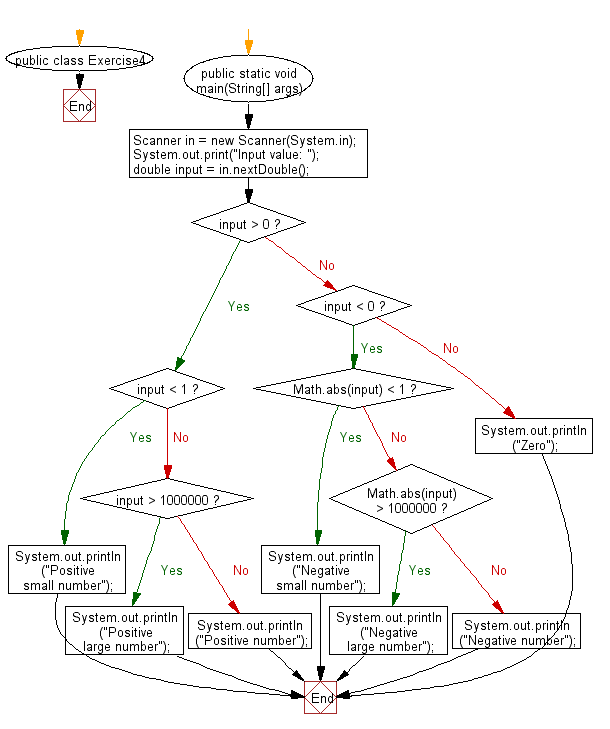﻿ Java exercises: Print a floating-point number in a specified format - w3resource# Java Conditional Statement Exercises: Print a floating-point number in a specified format

## Java Conditional Statement: Exercise-4 with Solution

Write a Java program that reads a floating-point number and prints "zero" if the number is zero. Otherwise, print "positive" or "negative". Add "small" if the absolute value of the number is less than 1, or "large" if it exceeds 1,000,000.

Test Data
Input value: 25

Pictorial Presentation:Sample Solution:

Java Code:

``````import java.util.Scanner;
public class Exercise4 {

public static void main(String[] args)
{
Scanner in = new Scanner(System.in);
System.out.print("Input value: ");
double input = in.nextDouble();

if (input > 0)
{
if (input < 1)
{
System.out.println("Positive small number");
}
else if (input > 1000000)
{
System.out.println("Positive large number");
}
else
{
System.out.println("Positive number");
}
}
else if (input < 0)
{
if (Math.abs(input) < 1)
{
System.out.println("Negative small number");
}
else if (Math.abs(input) > 1000000)
{
System.out.println("Negative large number");
}
else
{
System.out.println("Negative number");
}
}
else
{
System.out.println("Zero");
}
}
}
```
```

Sample Output:

```Input value: 25
Positive number
```

Flowchart:Java Code Editor:

Improve this sample solution and post your code through Disqus

What is the difficulty level of this exercise?

﻿